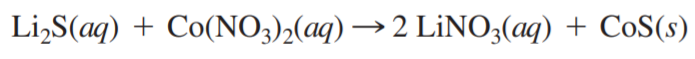×
Get Full Access to Chemistry: A Molecular Approach - 3 Edition - Chapter 4 - Problem 66e
Get Full Access to Chemistry: A Molecular Approach - 3 Edition - Chapter 4 - Problem 66e

×

# Consider the reaction: What volume of 0.150 M Li2SISBN: 9780321809247 1

## Solution for problem 66E Chapter 4

Chemistry: A Molecular Approach | 3rd Edition

• Textbook Solutions
• 2901 Step-by-step solutions solved by professors and subject experts
• Get 24/7 help from StudySoup virtual teaching assistantsChemistry: A Molecular Approach | 3rd Edition

4 5 1 240 Reviews
28
1
Problem 66E

Consider the reaction:What volume of 0.150 M Li2S solution is required to completely react with 125 mL of 0.150 M Co(NO3)2?

Step-by-Step Solution:
Step 1 of 3

Solution: Here, we are going to calculate the mass of alcohol circulating in the blood if the total blood volume is 5.0 L and BAC is 0.10. Step1: BAC or Blood Alcohol Concentration means units of grams of alcohol present per 100 mL of blood. For the problem given above, BAC = 0.10 which means, mass of alcohol present in 100 mL blood = 0.10 g Step2: Now, amount of alcohol present in 100 mL of blood = 0.10 g Therefore, amount of alcohol present in 5.0 L of blood = (0.10 g / 100 mL) X 5.0 L = 0.0010 g/mL X 5000 mL = 5.0 g Thus, required mass of alcohol circulating in the blood is 5.0 g. -------------------

Step 2 of 3

Step 3 of 3

##### ISBN: 9780321809247

Unlock Textbook Solution